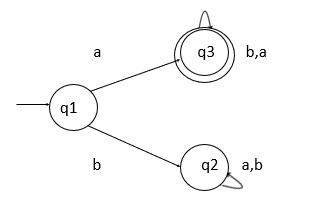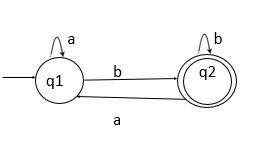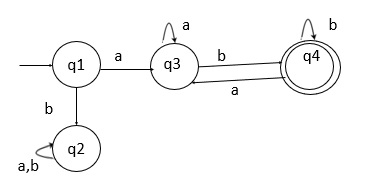# Explain the concatenation process in DFA

The concatenation process in the deterministic finite automata (DFA) is explained below −

If L1 and If L2 are two regular languages, their union L1 ∩ L2 will also be regular.

For example,

L1 = {an | n > O} and L2 = {bn | n > O}

L3 = L1 ∩ L2 = {an ∩ bn | n > O} is also regular.

In this property, we say that concatenation of two DFAs is also DFA.

## Problem

Design a DFA over an alphabet {a,b} where the string starts with ‘a’ and ends with ‘b’.

## Solution

There are two different types of languages are formed for a given condition, which are as follows −

• L1 = {a, aab, aabab, .......}
• L2 = {b, bbab, bbabab, .......}

In L1, starting element is ‘a’ and

L2, the ending element is ‘b’.

Language L1

It starts with ‘a’

L1 = {a, aab, aabab, .......}

The state transition diagram for L1 is as follows −In the above state transition diagram, q1 is the initial state.

Each state in DFA has to generate a transition on both inputs

So,

• q1 on ‘a’ goes to q3 and reaches its final state {a}.

• q1 on ‘b’ goes to q2 and reaches a dead state.

• q3 on ‘a’ goes to q3 reaching the final state generating a string from the start state {a,ab,aba,aab,abb,……} satisfies the condition that each string starts with ‘a’.

• q2 is a dead state.

Language L2

It ends with ‘b’

L2 = {b, bbab, bbabab, .......}

The state transition diagram for L2 is as follows −Initial state q1 : q1 on ‘a’ goes to q1

q1 on ‘b’ goes to q2

Once we generate the strings {ab,abb,abab,……}, it satisfies the condition that every string in the language ends with ‘b’.

Concatenate L1 and L2

When we concatenate L1 and L2, we get the following result −

L= L1 ∩ L2 = L1.L2 = {ab, aab, abb, aaab, ...}

The state transition diagram after the concatenation of L1 and L2 is given below −